# How To Solve A Simple Numeric Problem Using Computers and JavaScript

## The problem

Let's say I ask you to fix a number to make this equation true

Of course you might say - ohh it is easy, just put "3" where the `_` is. What if I ask you to find numbers between 1 and 10 to make this equation true

You probably now have to try a few numbers to find at least one solution.

As the size of the equation and the number of unknown numbers grows, manually trying combinations of numbers becomes slower and slower. Why not let the computers do this for us? Let me teach you how to program your browser to solve problems like this almost instantly.

## The environment

To find numbers we will use JavaScript programming language that runs in every browser (and even on the browsers on the phones). You can take any browser window, press `Options + Cmd + I` keys at the same time (I am writing this is on Mac) or select "View / Development / Developer Tools". You should see the Developer Tools tab opened. We will be using the "Console" tab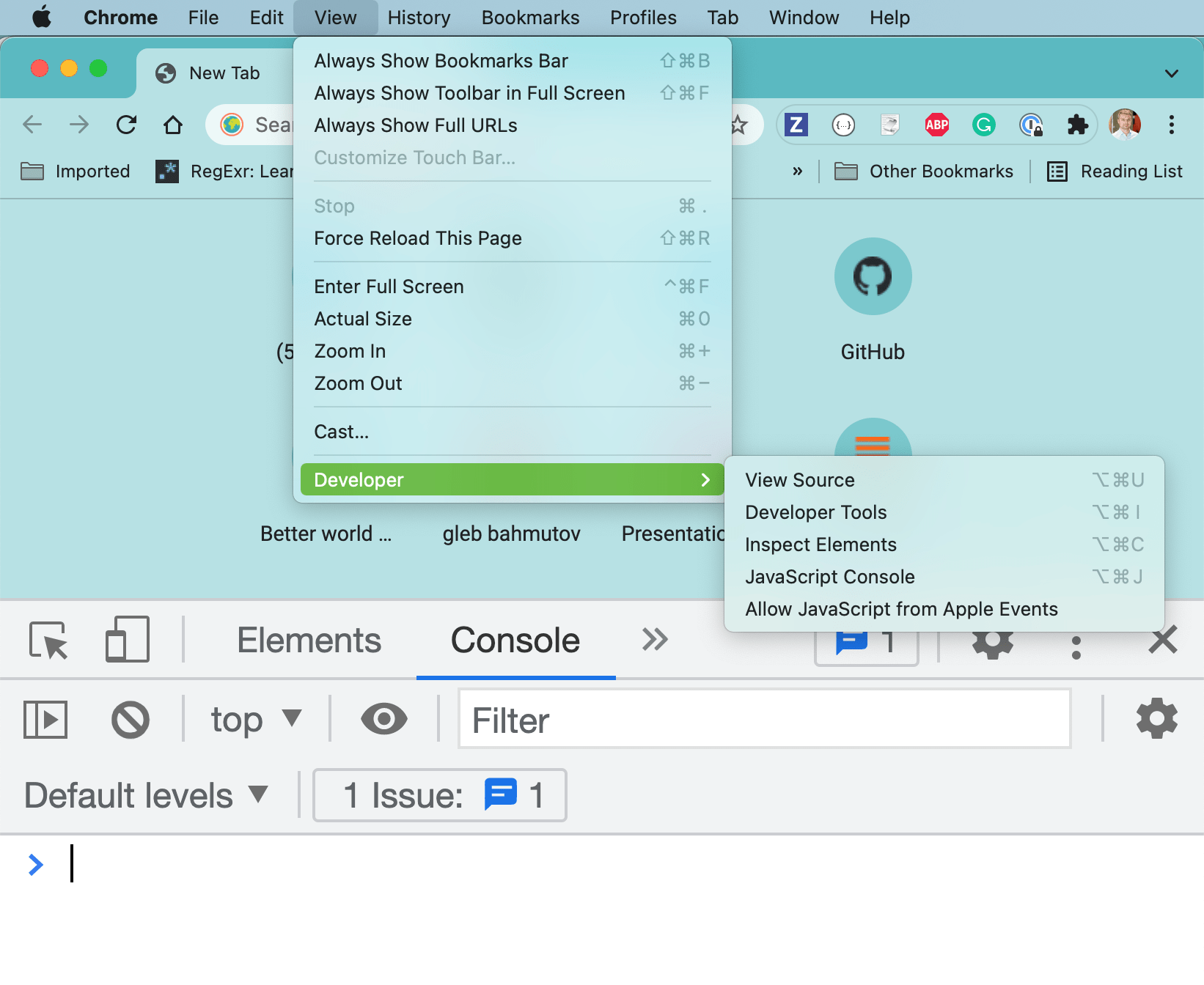You can open and close the Developer Tools (DevTools for short) at any moment in any browser window. Let's use the console to compute something for us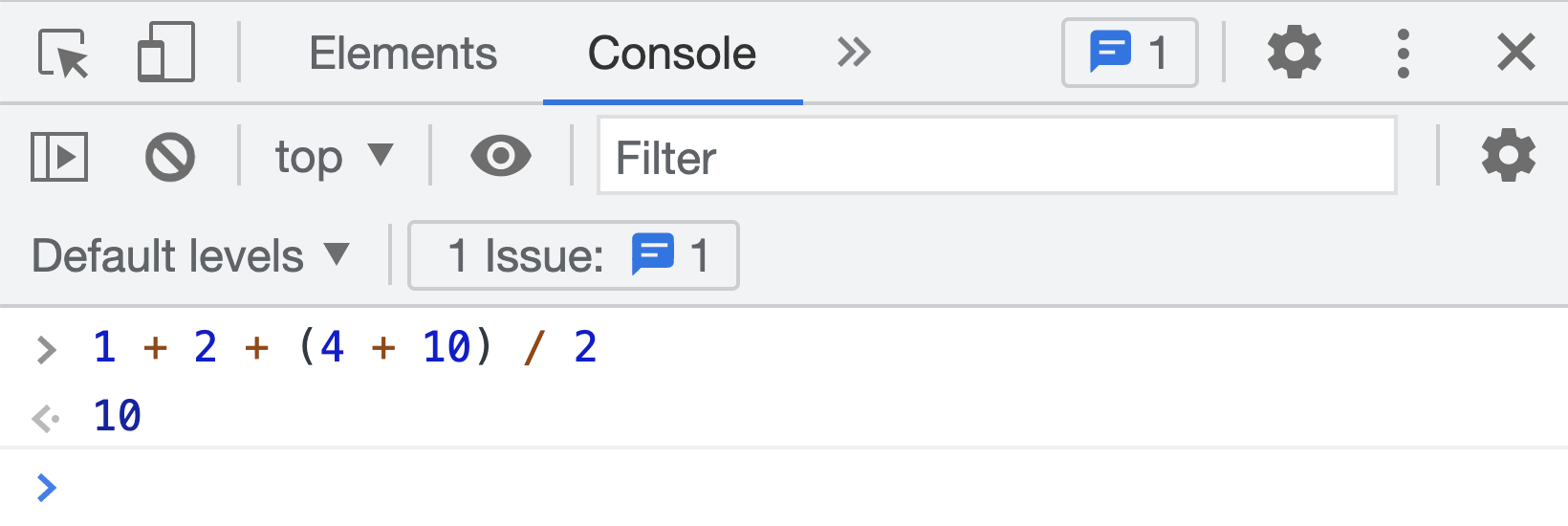## Comparing numbers

To find the solution to `_ = 3` let's try `1 = 3` in the DevTools console.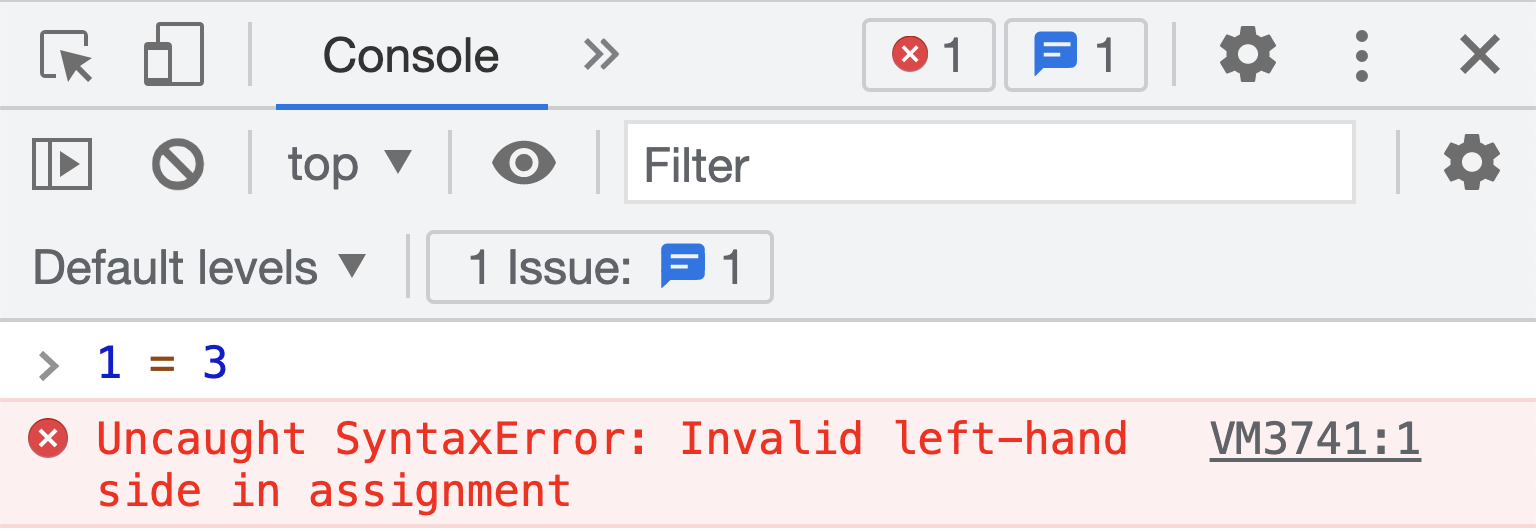Oops, the computer is complaining. JavaScript language uses the character `=` to assign a value to a variable, like `a = 10` and `x = 2`. We are only asking if `1` is equal to `3`. In JavaScript asking to compare to values has a special operator `===` that we will use.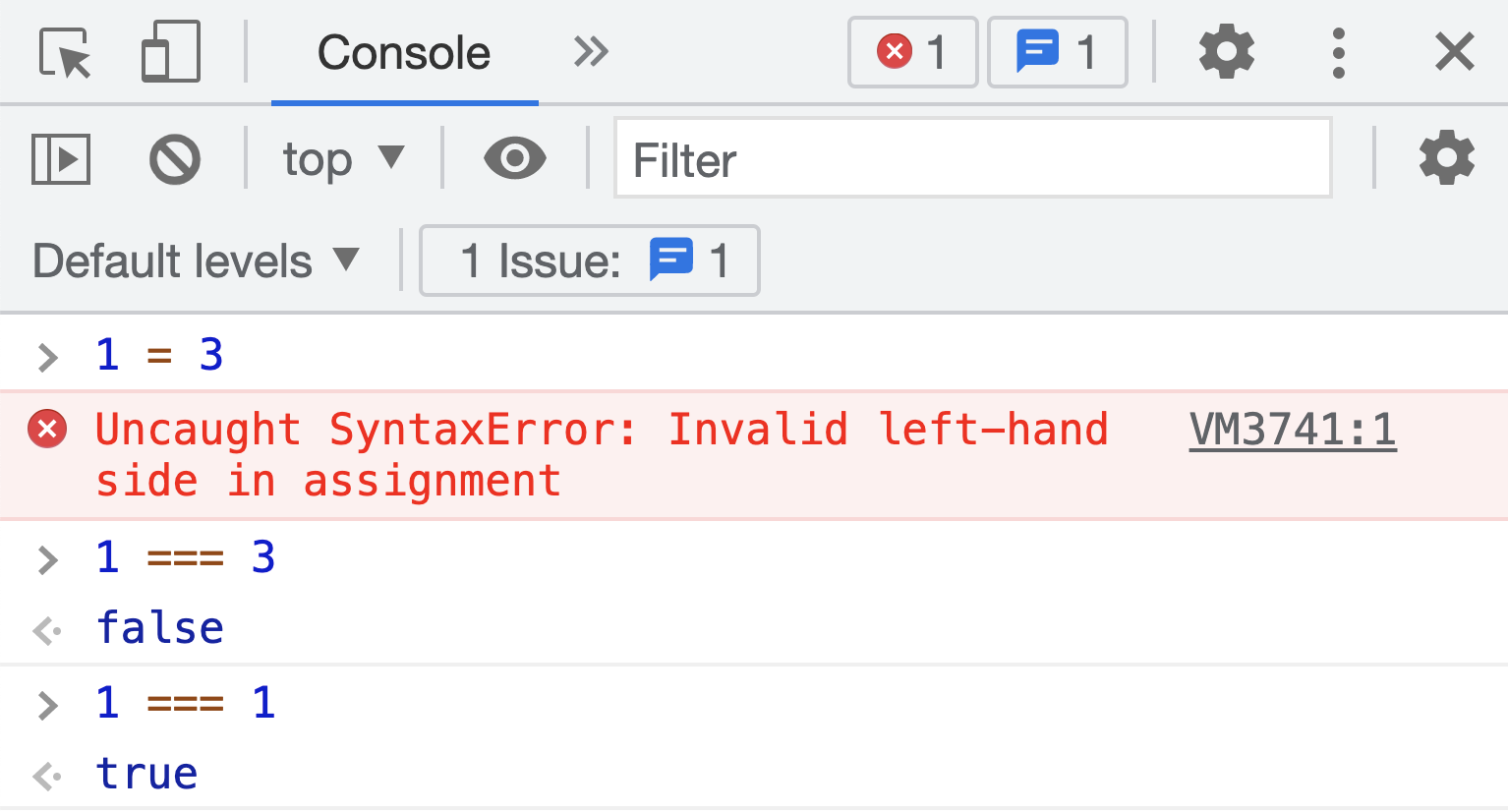When comparing numbers, we get back `true` or `false`.

## Printing

If the browser is computing something, it needs to print the results, and maybe print messages during the steps. To print something in the DevTools console, we can use the `console.log` function.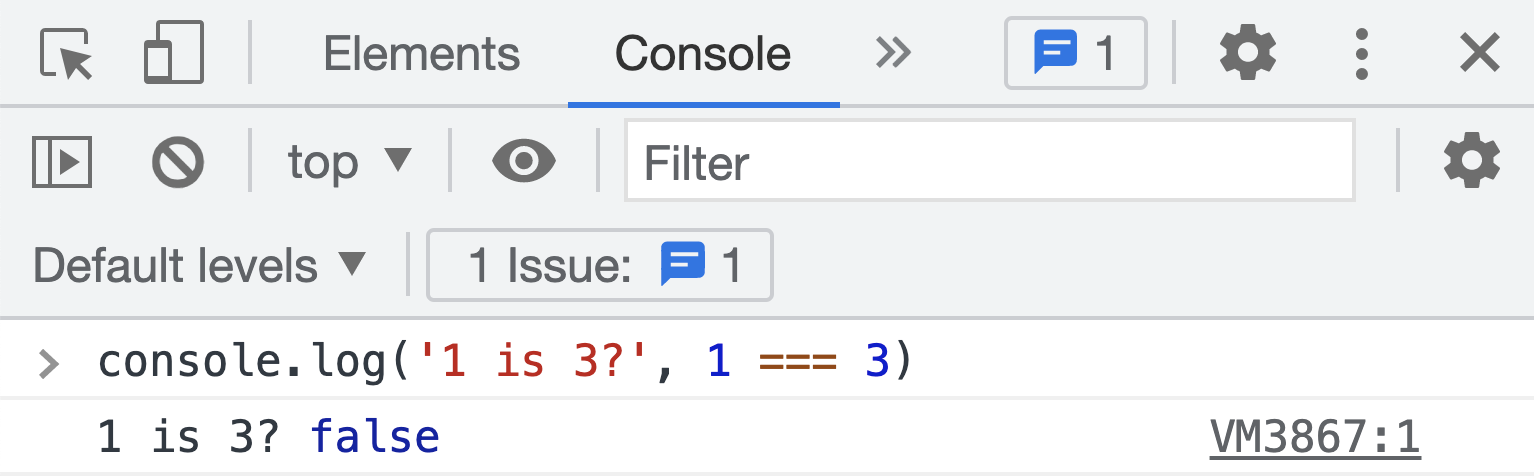You can pass one or multiple arguments to print. For example, we can compare numbers and print if one is larger or smaller than another.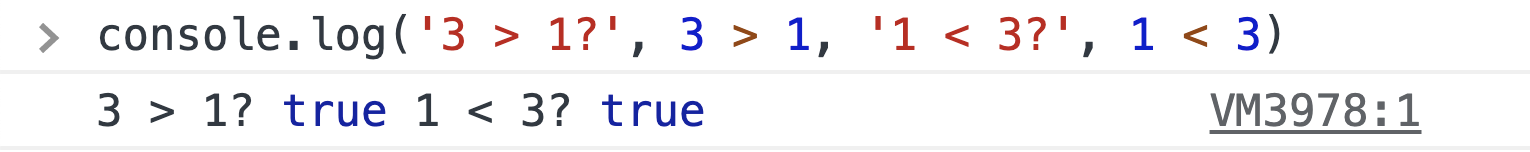## Variables

When solving `_ = 3` we need to try different values in place of `_`. Instead of `_` let's call the unknown value "x". We are trying to solve `x = 3` after all. In JavaScript, we can create `x` which called a variable. We can assign this variable (which is like an empty box) different values, then compare the current value to 3. Here is how it looks in DevTools: we are using `let x` to create a variable, `=` operator to put a value into `x`, and `===` to compare the current value of the variable to another value.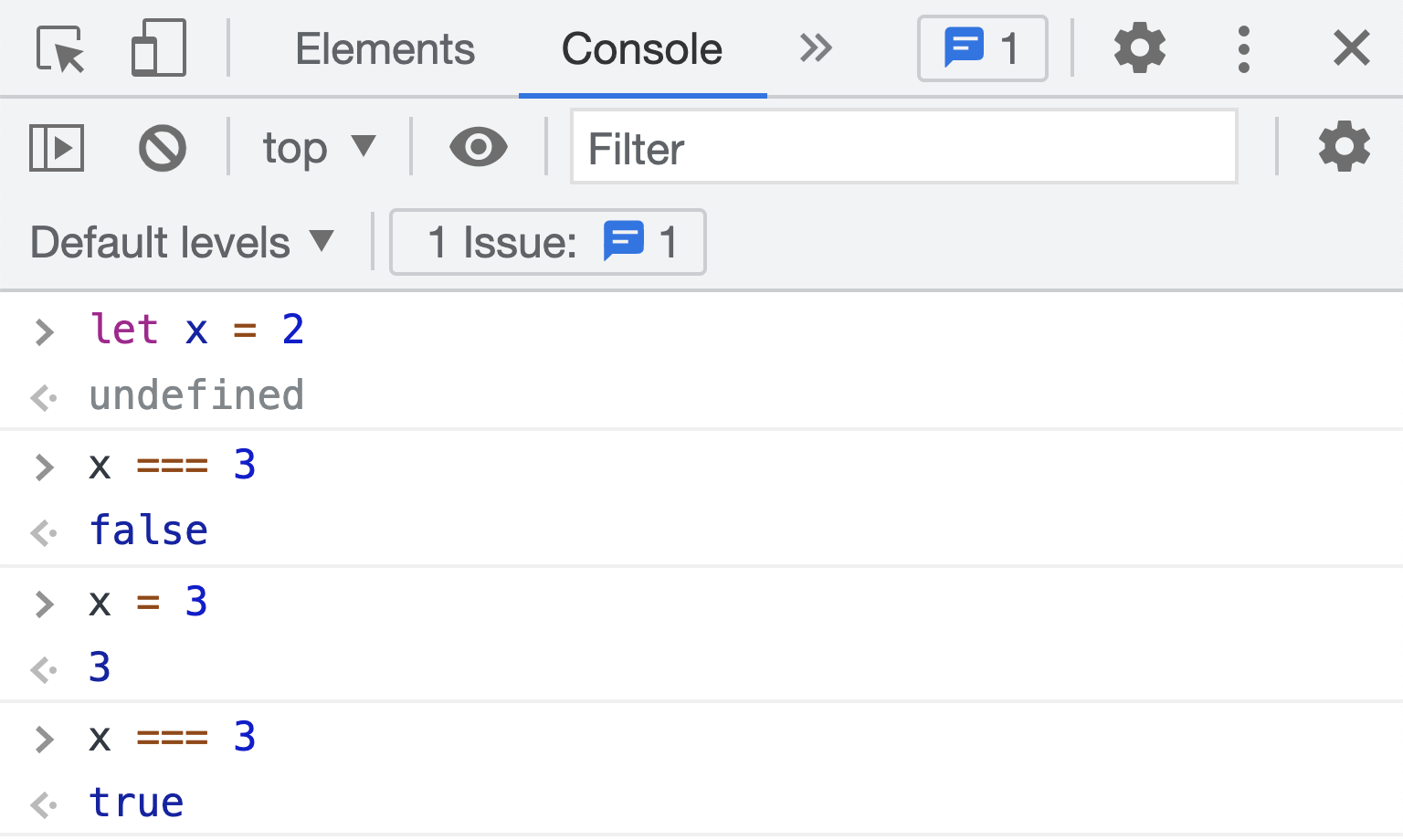We can explicitly print the comparison result using `console.log` making it very clear what is going on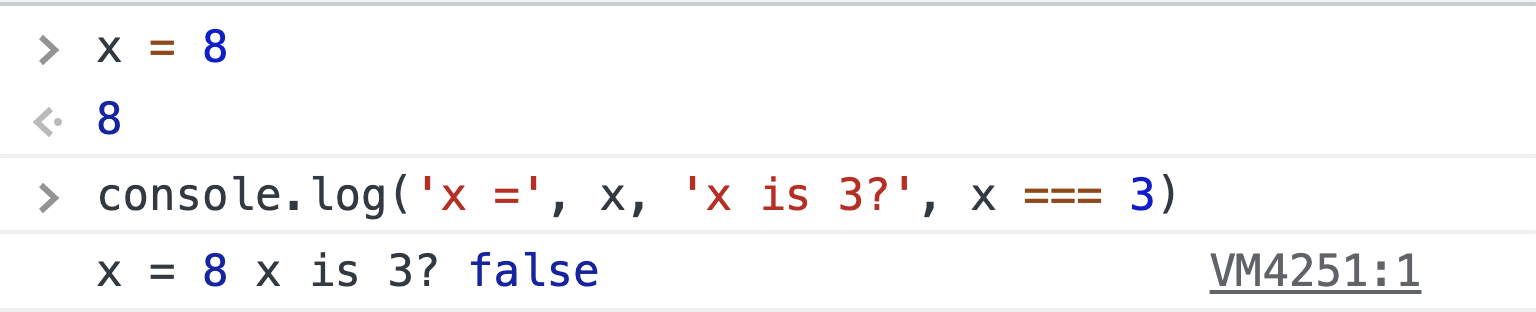To save space we can assign the value and compare it on one line, we just need to separate the assignment and the print statements using the special `;` to avoid JavaScript confusing them.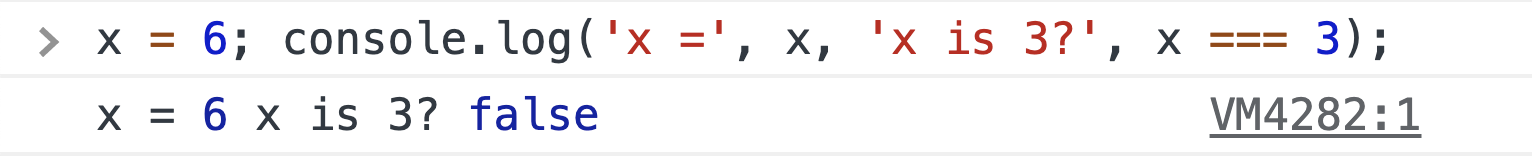## Functions

We will be comparing `x` to `3` multiple times while checking if we have found the solution. We can create a little reusable piece of code that takes `x` and tells us if the equation is true or false. The reusable pieces of code are called functions and have a special syntax.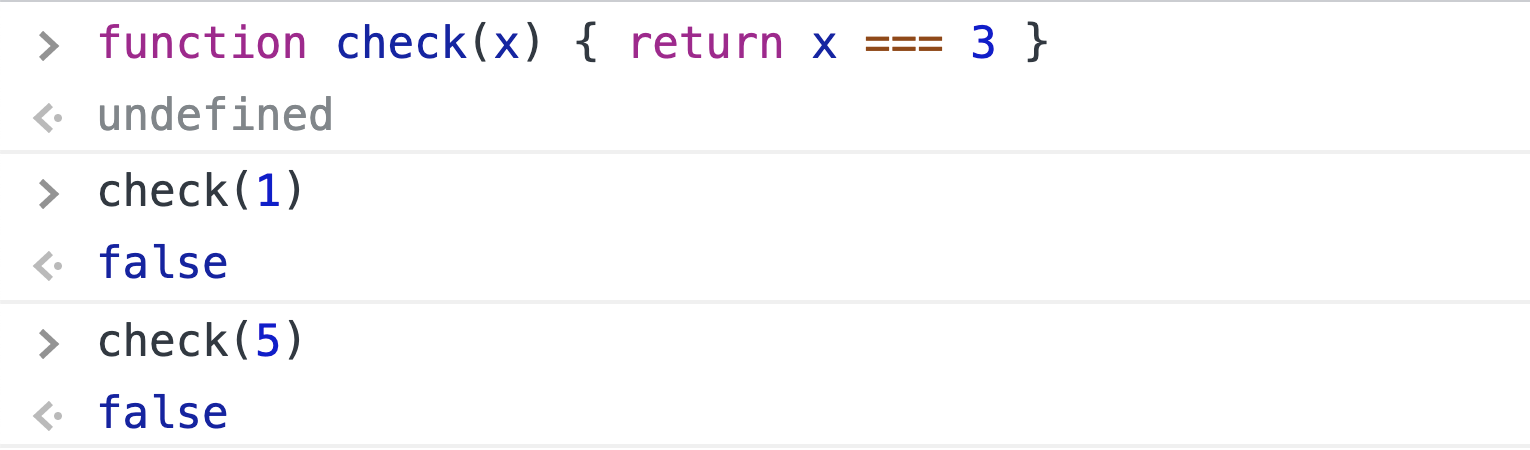Inside the function, `x` variable is equal to whatever we passed in the first argument place when we called `check`. When we called it `check(1)` inside the function `x` was 1. When we called it `check(5)` the value `x` was 5. Even if there is a variable `x` outside the function, the function does not care - it uses its internal value of the argument.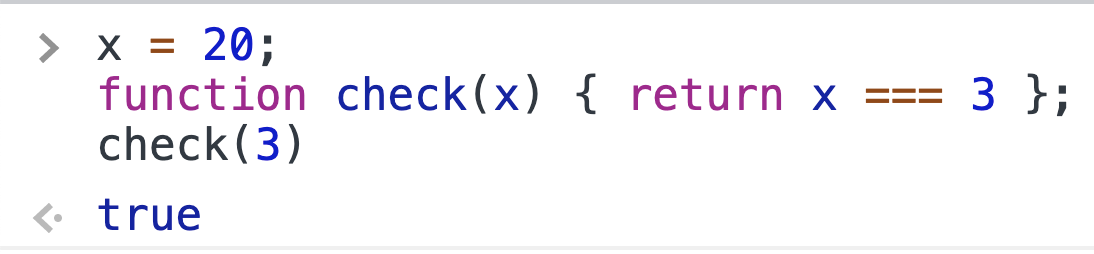Note how in the DevTools you can type multiple lines before executing them. Use `Shift + Enter` to add one more line. Use `Enter` key to run the JavaScript.

## Loops

Ok, so let's run all values from 1 to 10 through our function `check` and print the result.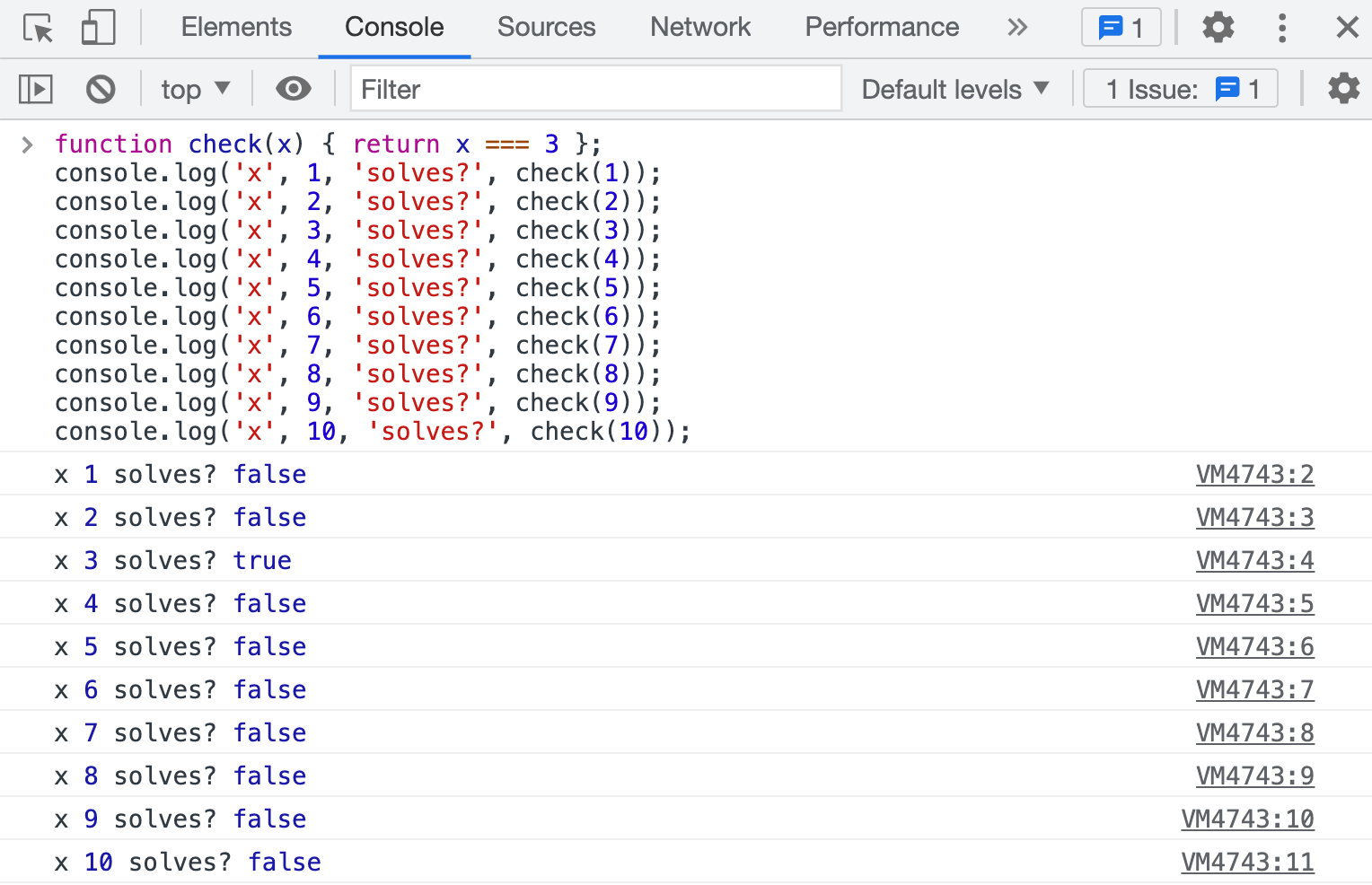Ughh, so verbose, and the only difference is the value we used to call `check(...)`. Let's remove the duplicate code. We can use a loop to execute the same statement again and again. The way to write this in JavaScript is to use another counter variable and specify its initial and final value and how to increment the variable after each iteration.

We have declared a new variable using `let k` just too keep track of the iteration. We gave it a starting value `let k = 1` and we will continue executing all code inside the curly braces `{ ... }` as long as the value of `k` is less than 11. Each iteration we will increment `k` by 1 using the special operator `+=`. Let's put our `check(...)` statement inside the loop.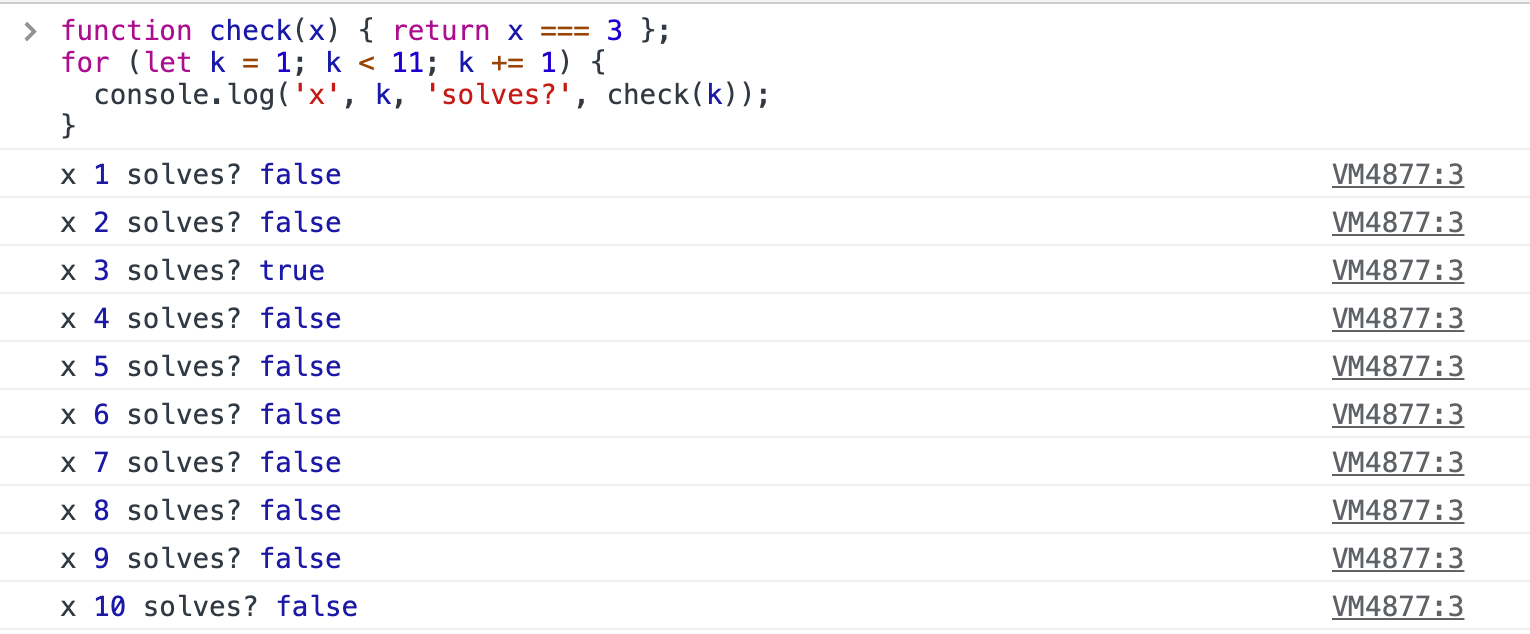Each iteration we call `check(...)` with the current value of the variable `k`. The function `check(x)` takes the passed value like 1, then 2, then 3, ... and puts it into its argument variable `x` and computes the result and returns it to be printed using `console.log`.

## Multiple loops

Let's say we are trying to solve an equation with multiple unknown values like `_ + 3 = _ * 2`. Our check function will need to expect 2 variables.

And we will need to try values from 1 to 10 for variable `x`. And for each `x` we need to try values from 1 to 10 for variable `y`. We can nest loops and just try different values; that's why it is called brute force approach. The computers can do billions of such calculations each second, so we don't have to worry about how long it takes to solve such problems. We expect 100 total checks (10 values of x * 10 values of y for each x):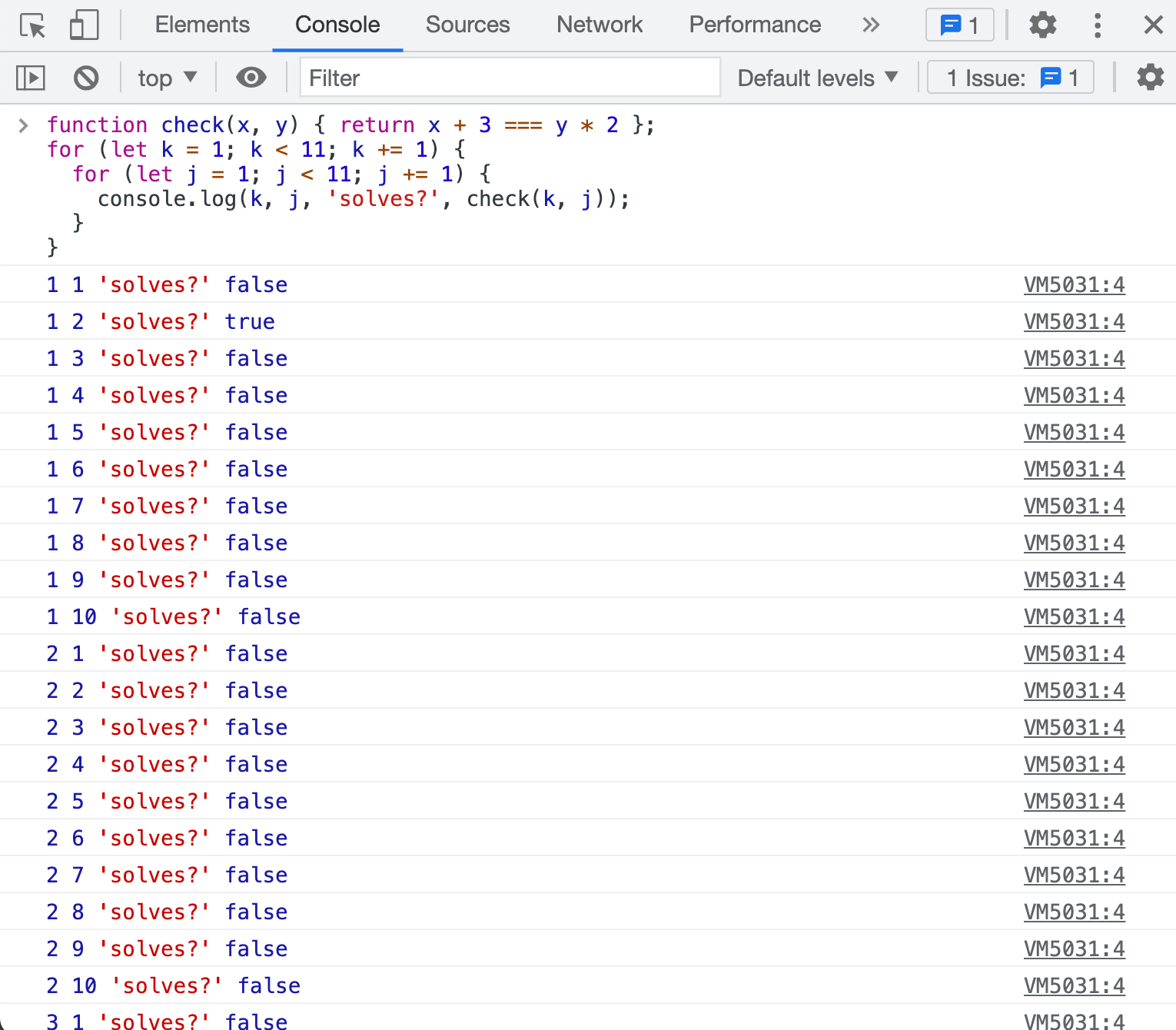It is very common to use variable names like `k`, `j`, `i` for loop counters, and `x`, `y` for unknown values that we are trying to find.

## Branches

In the image above, most of the 100 pairs return `false`, so let's print only the solutions. We can use the built-in JavaScript `if` operator

If there is nothing to do for the `else` branch, we can omit it

Here are our solutions found by iteration and only printing the ones where `check(...)` returns true.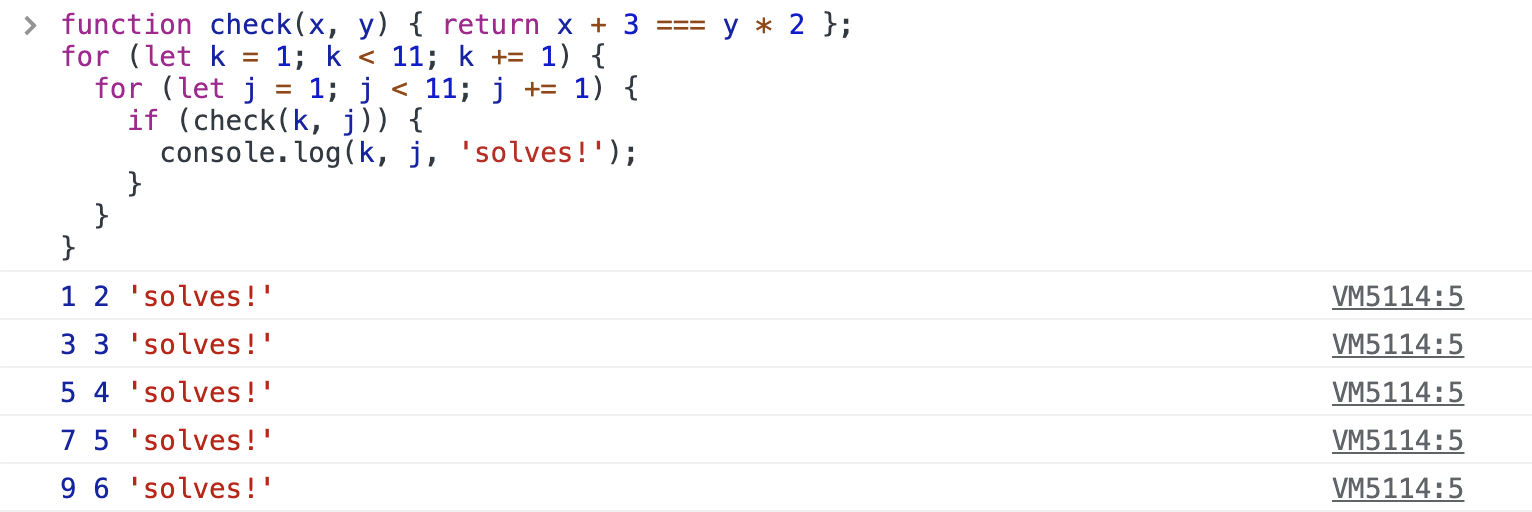## Variable names

JavaScript variables can be full words, and it is a good practice to use descriptive variable names that make it clear what the variable is holding inside.

On the other hand, if you have a lot of unknowns in your equation, you can use variable names with indices like `x1`, `x2`, `x3`, `x4` instead of one letter `x`, `y`, `v`, `w`. In the screenshot below I used `x1` and `x2` for unknowns and `k1` and `k2` for iteration counter variables.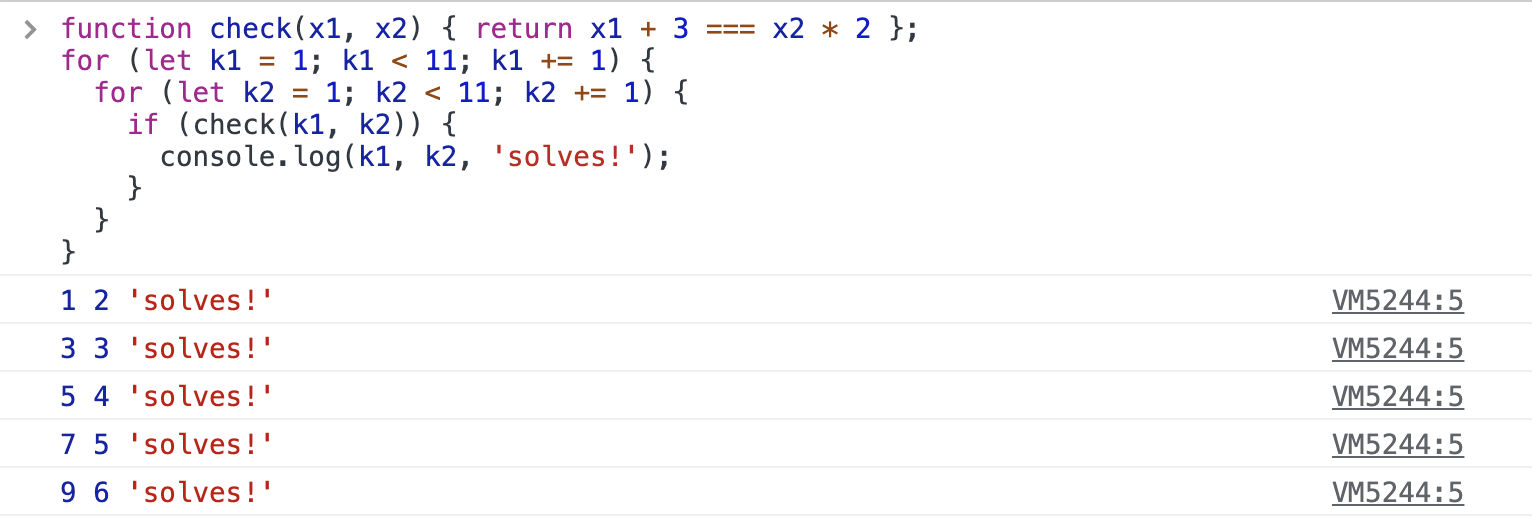## Challenges

Try to solve in your browser DevTools the following problems

1. what is the value of `(1 - 100) * 5 + 1234 + 588/2`.
1. Is it larger or smaller than the value of the expression `56 * 7`?
2. put the value of the first expression into the variable `left` and the value of the second expression into the variable `right` and print to the console both variables
2. print the word "Bot" 25 times in a row
3. find two numbers so that `x * 15 = 5 + y`. Each unknown number can be between 1 and 100
4. print "yes!!!" if the expression `45 * 19 * 22` is larger than 10000 and print "No :(" otherwise
5. find the number that solves the triple equality `x + 6 = 94 - 10 * x = 2 * x - 2`
6. iterate over numbers from 1 to 300 in increments of 6 and print the numbers. Your first few numbers should be 1, 7, 13, ...